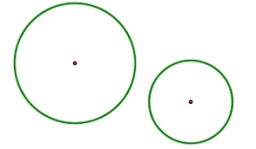# Determine 44221

For circles k1 (S1,4cm) and k2 (S2,3cm) and it holds that | S1S2 | = 8cm. Determine the distance between the circles K1 and K2.

x =  1 cm

### Step-by-step explanation:Did you find an error or inaccuracy? Feel free to write us. Thank you!

Tips for related online calculators
Line slope calculator is helpful for basic calculations in analytic geometry. The coordinates of two points in the plane calculate slope, normal and parametric line equation(s), slope, directional angle, direction vector, the length of the segment, intersections of the coordinate axes, etc.
Do you want to convert length units?

#### Grade of the word problem:

We encourage you to watch this tutorial video on this math problem: